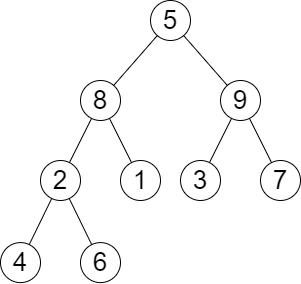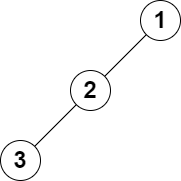2583. Kth Largest Sum in a Binary Tree
Medium
223
5

You are given the `root` of a binary tree and a positive integer `k`.

The level sum in the tree is the sum of the values of the nodes that are on the same level.

Return the `kth` largest level sum in the tree (not necessarily distinct). If there are fewer than `k` levels in the tree, return `-1`.

Note that two nodes are on the same level if they have the same distance from the root.

Example 1:```Input: root = [5,8,9,2,1,3,7,4,6], k = 2
Output: 13
Explanation: The level sums are the following:
- Level 1: 5.
- Level 2: 8 + 9 = 17.
- Level 3: 2 + 1 + 3 + 7 = 13.
- Level 4: 4 + 6 = 10.
The 2nd largest level sum is 13.
```

Example 2:```Input: root = [1,2,null,3], k = 1
Output: 3
Explanation: The largest level sum is 3.
```

Constraints:

• The number of nodes in the tree is `n`.
• `2 <= n <= 105`
• `1 <= Node.val <= 106`
• `1 <= k <= n`
Accepted
19K
Submissions
40.7K
Acceptance Rate
46.6%

Seen this question in a real interview before?
1/4
Yes
No

Discussion (0)

Related Topics